python for data analysis Archives - DexLab Analytics | Big Data Hadoop SAS R Analytics Predictive Modeling & Excel VBA

## Theory of Estimation Part-I: The Introduction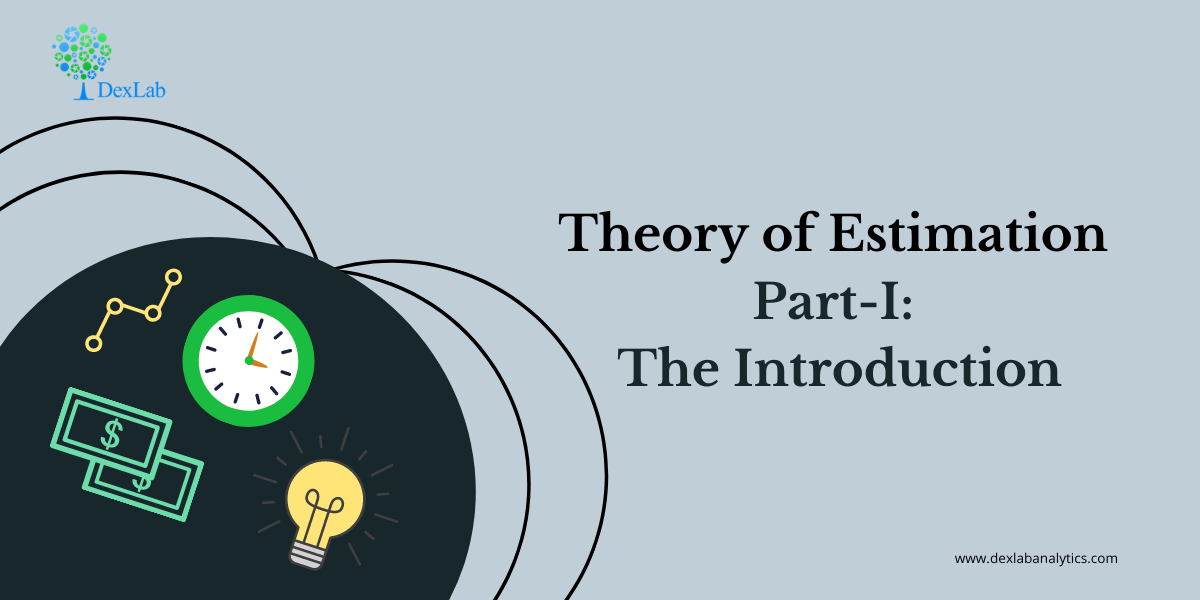The theory of estimation is a branch in statistics that provides numerical values of the unknown parameters of the population on the basis of the measured empirical data that has a random component. This is a process of guessing the underlying properties of the population by observing the sample that has been taken from the population. The idea behind this is to calculate and find out the approximate values of the population parameter on the basis of a sample statistics.

Population:- All the items in any field of inquiry constitutes to a “Population”. For example all the employees of a factory is a population of that factory and the population mean is represented and the size of the population is represented by N.

Sample:- Selection of few items from the population constitutes to a sample and the mean of the sample is represented by  and the sample size is represented by n

Statistics:- Any statistical measure calculated on the basis of sample observations is called Statistic. Like sample mean, sample standard deviation, etc.

Estimator:- In general estimator acts as a rule, a measure computed on the basis of the sample which tells us how to calculate the values of the estimate. It is a functional form of all sample observations prorating a representative value of the collected sample.

Suppose we have a random sample x_1,x_2,…,x_n on a variable x, whose distribution in the population involves an unknown parameter. It is required to find an estimate of on the basis of sample values.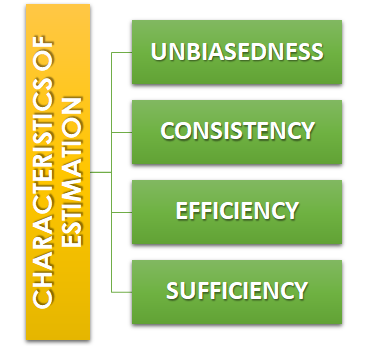Unbiasedness:-A statistic t is said to be an unbiased estimator if E(β ̂)= βi.e. observed value is equal to the expected value. In case E(β ̂)≠ β then the estimator is biased estimator.

Consistency:- One of the most desirable property of good estimator is that its accuracy should increase when the sample becomes larger i.e. the error between the expected value and the observed value reduces as the size of the sample increases E(β ̂ )- β=0

Efficiency:-An estimator is said to be an efficient estimator if it has the smallest variance compared to all the consistent and unbiased estimators. If consistent estimator exists whose sampling variance is less than that of any other consistent estimator, it is said to be “most efficient”; and it provides a standard for the measurement of ‘efficiency’ of a statistic.Sufficiency:- An estimator is said to be sufficient if it contains all information in the sample about .

At the end of this discussion, hopefully, you have learned what theory of estimation is. Watch the video tutorial attached below to learn more about this. DexLab Analytics is a data science training institute in gurgaon, that offers advanced courses. Follow the blog section to access more informative posts like this.

.

## An Introductory Guide to NumPy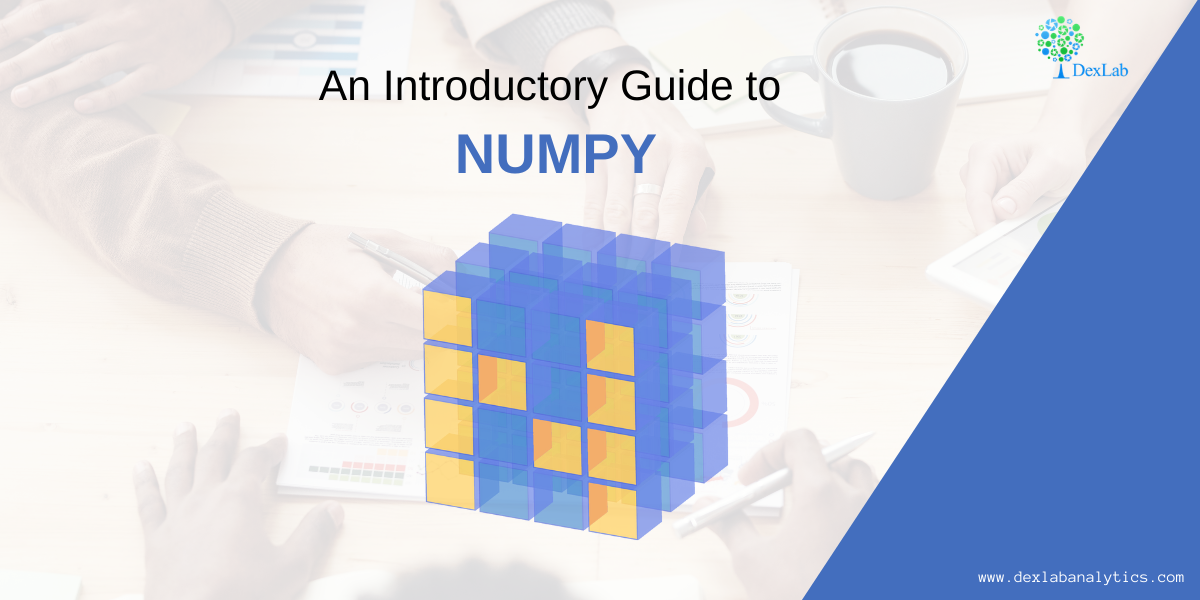NumPy also known as numerical python, is a library consisting of multidimensional array objects and a collection of routines for processing those arrays. Using NumPy, mathematical and logical operations on arrays can be performed without it which was not possible. For example-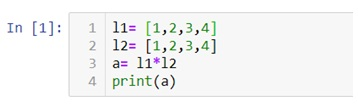Multiplication of two lists will cause an error as a data structure like lists, tuple, dictionaries and sets do not allow mathematical operations.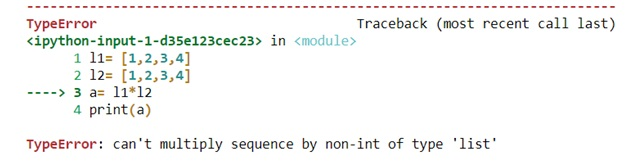Therefore we need NumPy to covert our data structures like lists into 1d, 2d, 3d or nd arrays so that mathematical operations can be performed. U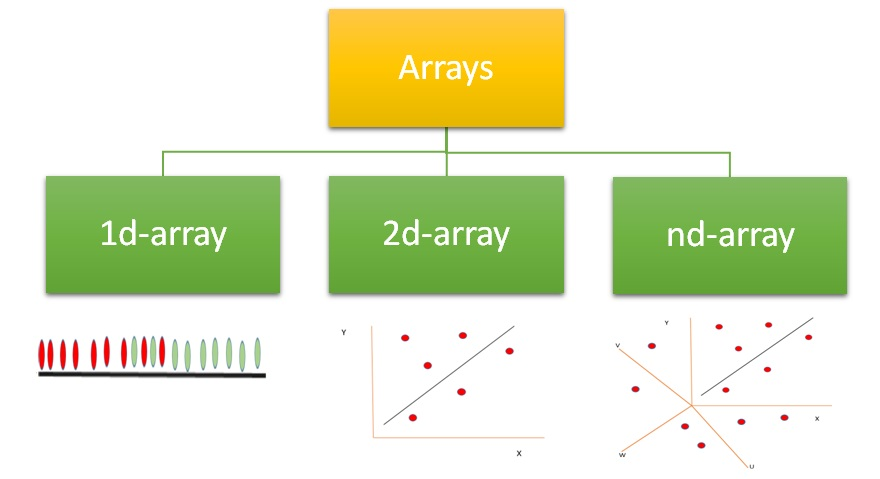We can use .array() methods to create these arrays.

Now let’s check out few examples and also perform few mathematical operations to have a better understanding.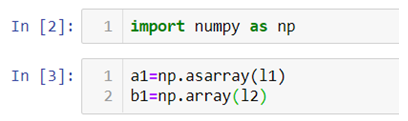Arrays can be created using lists, tuples and dictionaries as you can see in the above example.

Now for 2-d arrays recall that we can also make list of lists. Let’s use that to create 2d-arrays.

2d-arrays can also be created using tuples.

Remember that we are not using these as matrices because matrix multiplication is an entirely different thing we are just trying to perform mathematical operations which were otherwise not possible.

#### Random Module

Numpy also has various ways with which we can create array of random numbers which then can be used in number of ways like generating a data for practice purposes or for building beautiful graphs for a presentation.

Given below is a list of type of random numbers you can generate

.rand() :- This particular method helps you generate uniformly distributed random numbers i.e. numbers between 0 and 1 where each number between 0 and 1 will have equal probability to be in the sample dataset.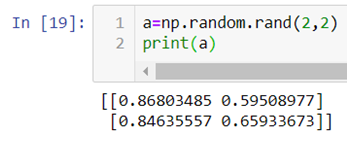The above code generates a 2d-array with values between 0 and 1.

.randn():- This method generates normally distributed random numbers i.e. numbers between -3 and +3 where mean=median=mode and ploted gives a bell shaped curve.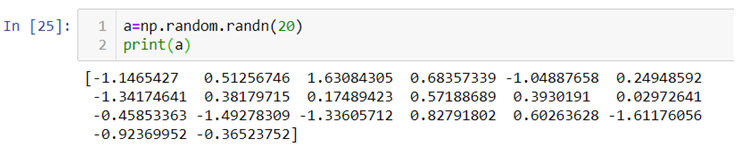Here the 20 random numbers are generated ranging between -3 and + 3.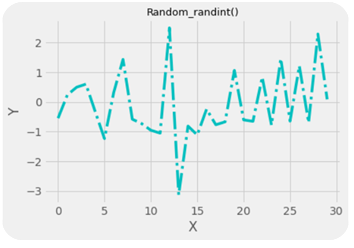Note:- Remember that the data is randomly picked from the normally distributed values between -3 and +3 so the graph is not bell shaped but the original data from which the values are being picked randomly is bell shaped with mean=median-mode.

.randint():-This method generates random integers between a given range.

So, with that we come to the end of the discussion on the Numpy. Hopefully it helped you understand Numpy, for more information you can also watch the video tutorial attached down this blog. DexLab Analytics offers machine learning courses in delhi. To keep on learning more, follow DexLab Analytics blog.

.

## Linear Regression Part II: Predictive Data Analysis Using Linear Regression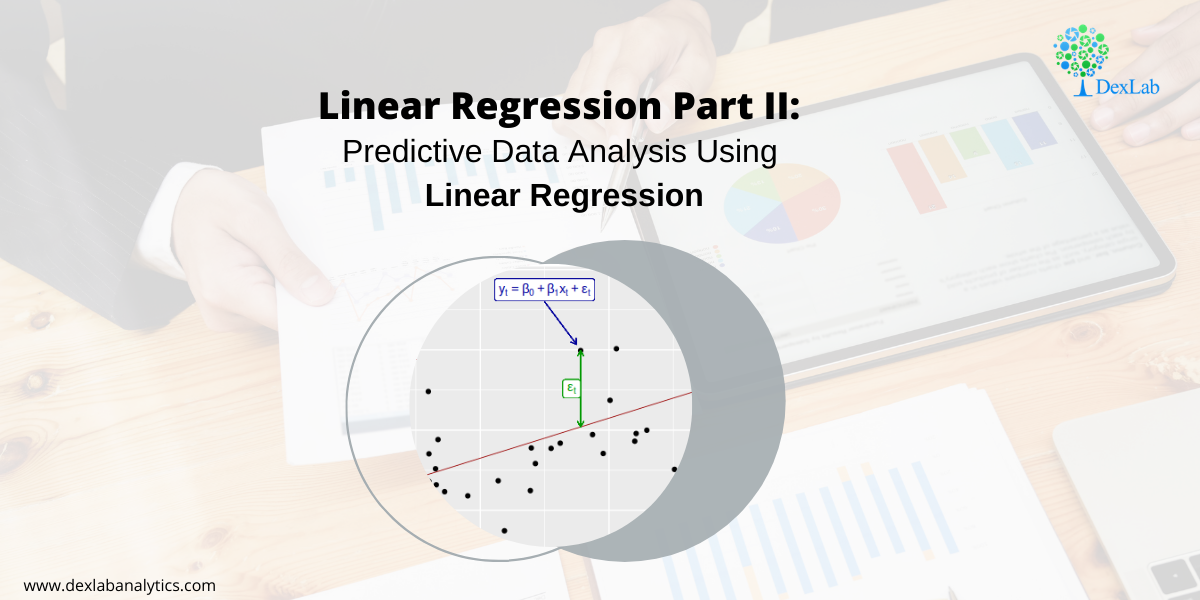In our previous blog we studied about the basic concepts of Linear Regression and its assumptions and let’s practically try to understand how it works.

Given below is a dataset for which we will try to generate a linear function i.e.

y=b0+b1Xi

Where,

y= Dependent variable

Xi= Independent variable

b0 = Intercept (coefficient)

b1 = Slope (coefficient)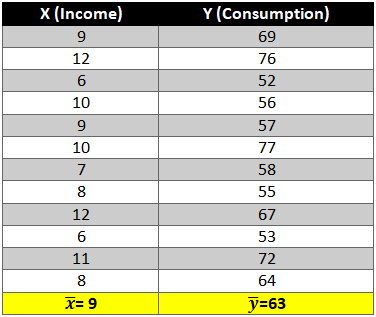To find out beta (b0& b1) coefficients we use the following formula:-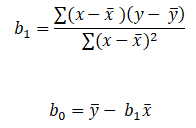Let’s start the calculation stepwise.

1. First let’s find the mean of x and y and then find out the difference between the mean values and the Xi and Yie. (x-x ̅ ) and (y-y ̅ ).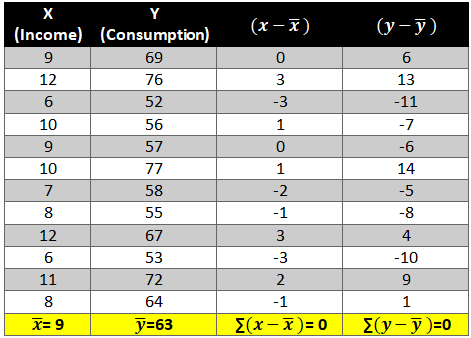2. Now calculate the value of (x-x ̅ )2 and (y-y ̅ )2. The variation is squared to remove the negative signs otherwise the summation of the column will be 0.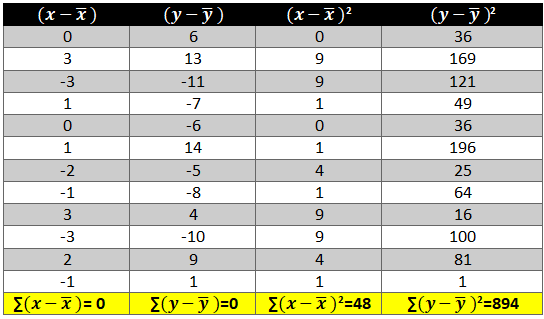3. Next we need to see how income and consumption simultaneously variate i.e. (x-x ̅ )* (y-y ̅ )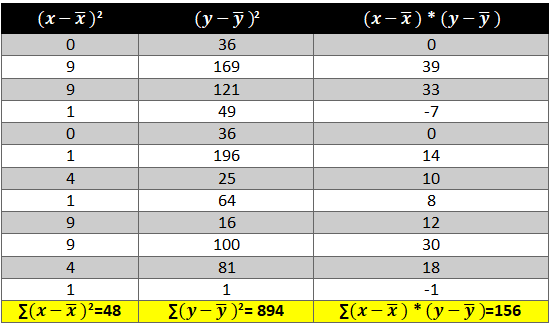Now all there is left is to use the above calculated values in the formula:-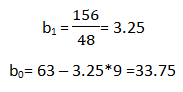As we have the value of beta coefficients we will be able to find the y ̂(dependent variable) value.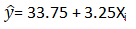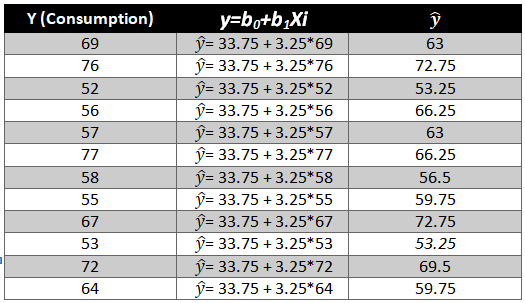We need to now find the difference between the predicted y ̂ and observed y which is also called the error term or the error.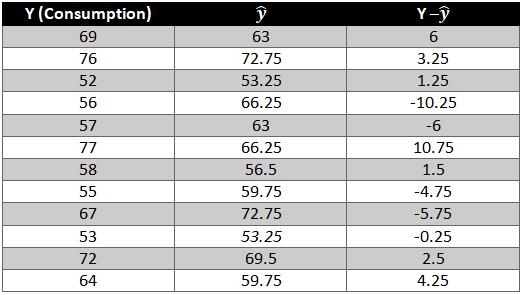To remove the negative sign lets square the residual.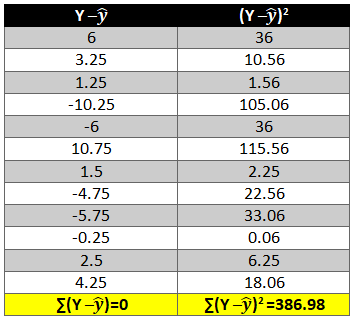What is R2 and adjusted R2 ?

R2 also known as goodness of fit is the ratio of the difference between observed y and predicted  and the observed y and the mean value of y.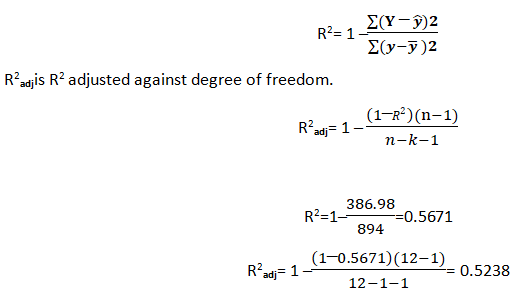Hopefully, now you have understood how to solve a Linear Regression problem and would apply what you have learned in this blog. You can also follow the video tutorial attached down the blog. You can expect more such informative posts if you keep on following the DexLab Analytics blog. DexLab Analytics provides data Science certification courses in gurgaon.

.

## An Introduction to Sampling and its Types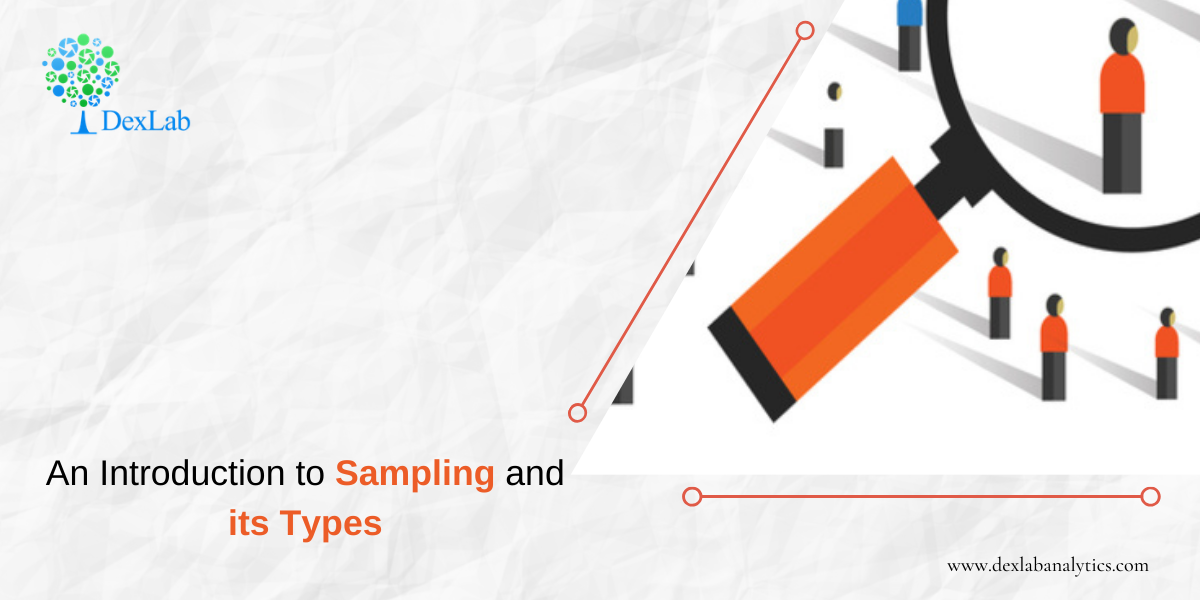Sampling is a technique in which a predefined number of observation is taken from a large population for the purpose of statistical analysis and research.

There are two types of sampling techniques:-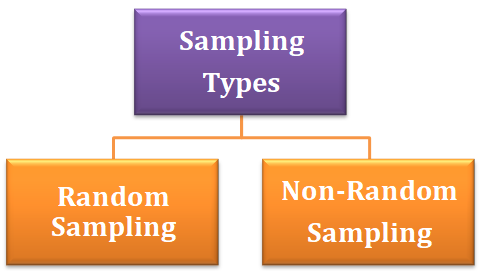#### Random Sampling

Random sampling is a sampling technique in which each observation has an equal probability of being chosen. This kind of sample should be an unbiased representation of the population.

#### Types of random sampling

1. Simple Random Sampling:- Simple random sampling is a technique in which any observation can be chosen and each observation has an equal probability of being selected.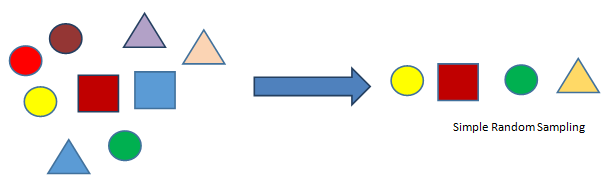2. Stratified Random Sampling:- In this sampling technique we create sub-group of the population with similar attributes and characteristics and then out of those sub-groups we then include each category in our sample with the probability of choosing each observation from the sub-group being equal.3. Systematic Sampling:- This is a sampling technique where the first observation is selected randomly and then every kth element is chosen randomly to be included in our sample.
k= 2, here the first observation is selected randomly and after that every second element is included in the sample.4. Cluster Sampling:- This is a sampling technique in which the data is grouped into small sub-groups called clusters with random categories and then from those clusters random observation is selected which then is included in the sample.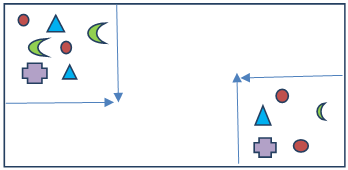Two clusters are created from which then random observation will be chosen to form the sample.

Non-Random Sampling :- It is a sampling technique in which an element of biasedness is introduced which means that an observation is selected for the sample on the basis of not probability but choice.

Types of non-random sampling:-

1. Convenience Sampling:- When a sample observation is drawn from the population based on how comfortable it is for you to take the observation it is called convenience sampling. For example when you have a survey sheet that is to be filled by students from all the departments of your college but you only ask your friends to fill the survey sheet.
2. Judgment Sampling:– When the sample observation drawn from the population is based on your professional judgment or past experience, it is called judgment sampling.
3. Quota Sampling:– When you draw a sample observation from the population that is based on some specific attribute, it is called quota sampling. For example, taking sample of people over and above 50 years.
4. Snow Ball Sampling:– When survey subjects are selected based on referral from other survey respondents, it is called snow ball sampling.

#### Sampling and Non-sampling errors

Sampling errors:- It occurs when the sample is not representative of the entire population.  For example a sample of 10 people with or without COVID-19 cannot tell whether or not the entire population of a country is COVID positive.

Non-sampling error:-This kind of error occurs during data collection. For example, during data collection if you falsely specified a name, it will be considered a non-sampling error.

So, with that this discussion on Sampling wraps up, hopefully, at the end of this you have learned what Sampling is, what are its variations and how do they all work. If you need further clarification, then check out our video tutorial on Sampling attached down the blog. DexLab Analytics provides the best data science course in gurgaon, keep following the blog section to stay updated.

.

## Hypothesis Testing: An Introduction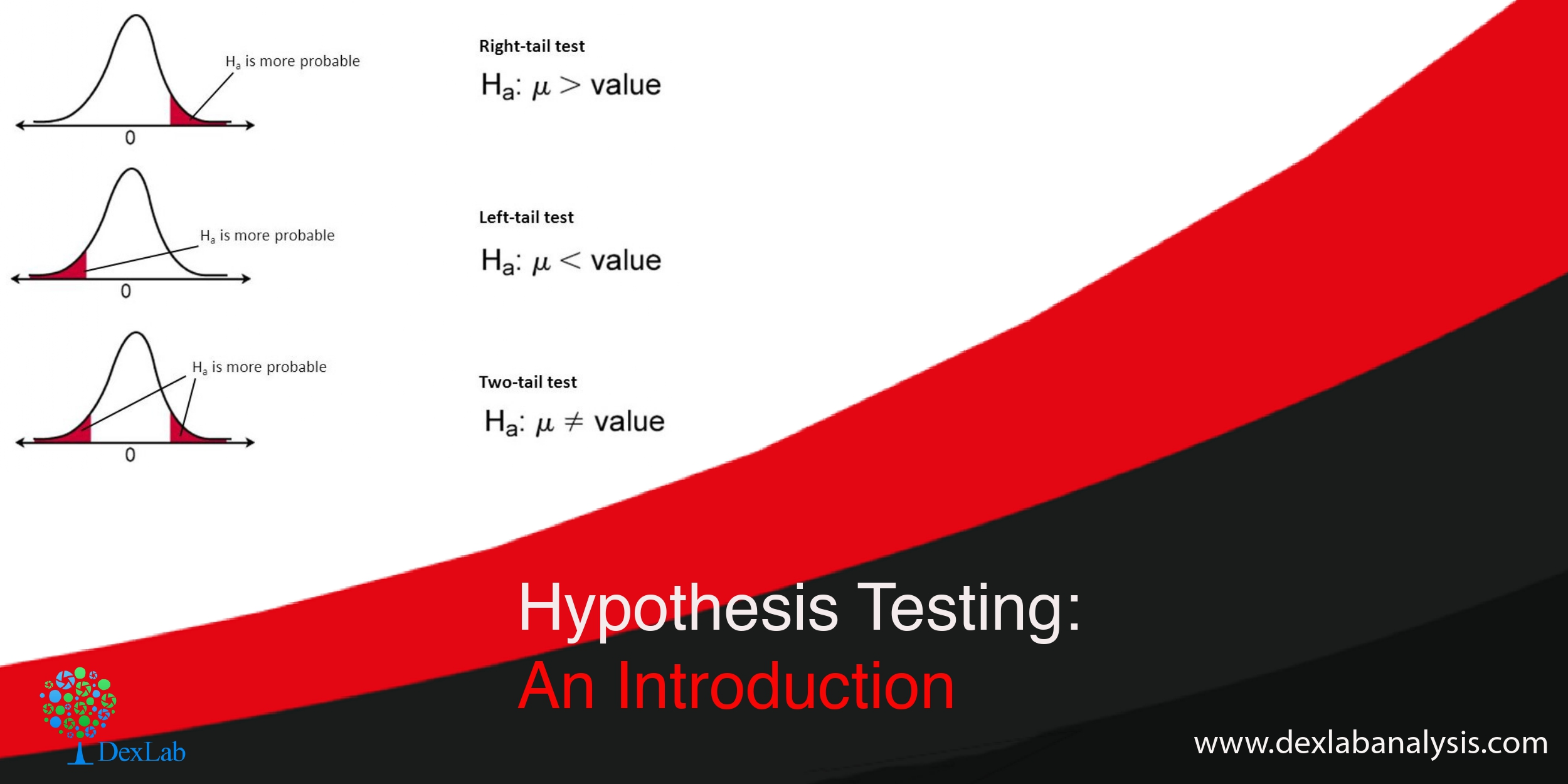You must be familiar with the phrase hypothesis testing, but, might not have a very clear notion regarding what hypothesis testing is all about. So, basically the term refers to testing a new theory against an old theory. But, you need to delve deeper to gain in-depth knowledge.

Hypothesis are tentative explanations of a principal operating in nature. Hypothesis testing is a statistical method which helps you prove or disapprove a pre-existing theory.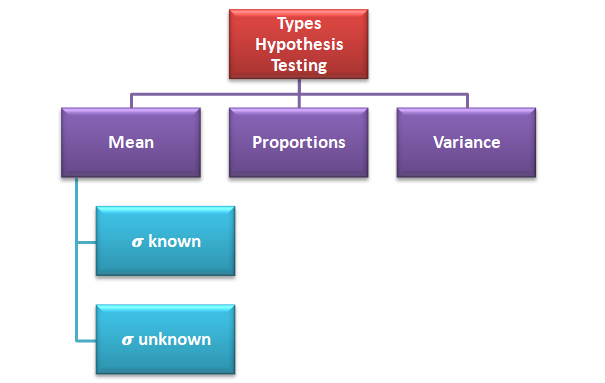Hypothesis testing can be done to check whether the average salary of all the employees has increased or not based on the previous year’s data, testing can be done to check if the percentage of passengers increased or not in the business class due to introduction of a new service and testing can also be done to check the differences in the productivity varied land.

#### There are two key concepts in testing of hypothesis:-

Null Hypothesis:- It means the old theory is correct, nothing new is happening, the system is in control, old standard is correct etc. This is the theory you want to check if is true or not. For example if a ice-cream factory owner says that their ice-cream contains 90% milk, this can be written as:-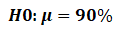Alternative Hypothesis:- It means new theory is correct, something is happening, system is out of control, there are new standards etc. This is the theory you check against the null hypothesis. For example you say that ice-cream does not contain 90% milk, this can be written as:-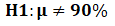#### Two-tailed, right tailed and left tailed test

Two-tailed test:- When the test can take any value greater or less than 90% in the alternative it is called two-tailed test ( H190%) i.e. you do not care if the alternative is more or less all you want to know is if it is equal to 90% or no.

Right tailed test:-When your test can take any value greater than 90% (H1>90%) in the alternative hypothesis it is called right tailed test.

Left tailed test:-When your test can take any value less than 90% (H1<90%) in the alternative hypothesis it is called left tailed test.

#### Type I error and Type II error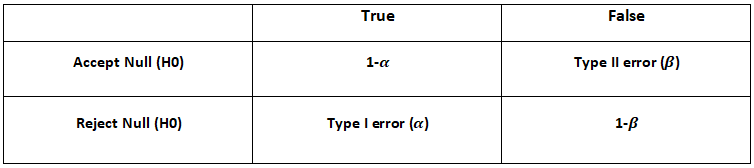->When we reject the null hypothesis when it is true we are committing type I error. It is also called significance level.

->When we accept the null hypothesis when it is false we are committing type II error.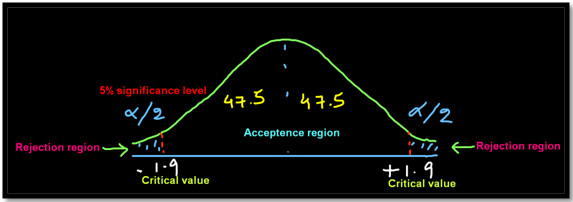#### Steps involved in hypothesis testing

1. Build a hypothesis.
2. Collect data
3. Select significance level i.e. probability of committing type I error
4. Select testing method i.e. testing of mean, proportion or variance
5. Based on the significance level find the critical value which is nothing but the value which divides the acceptance region from the rejection region
6. Based on the hypothesis build a two-tailed or one-tailed (right or left) test graph
7. Apply the statistical formula
8. Check if the statistical test falls in the acceptance region or the rejection region and then accept or reject the null hypothesis

Example:- Suppose the average annual salary of the employees in a company in 2018 was 74,914. Now you want to check whether the average salary  of the employees has increased or not in 2019. So, a sample of 112 people were taken and it was found out  that the average annual salary of the employees in 2019 is 78,795. σ=14.530.

We will apply hypothesis testing of mean when  known with 5% of significance level.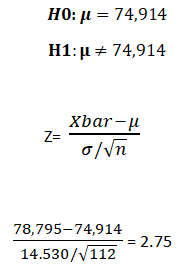The test result shows that 2.75 falls beyond the critical value of 1.9 we reject the null hypothesis which basically means that the average salary has increased significantly in 2019 compared to 2018.

So, now that we have reached at the end of the discussion, you must have grasped the fundamentals of hypothesis testing. Check out the video attached below for more information. You can find informative posts on Data Science course, on Dexlab Analytics blog.

.

## Linear Regression Part I: A Comprehensive Guide to Linear Regression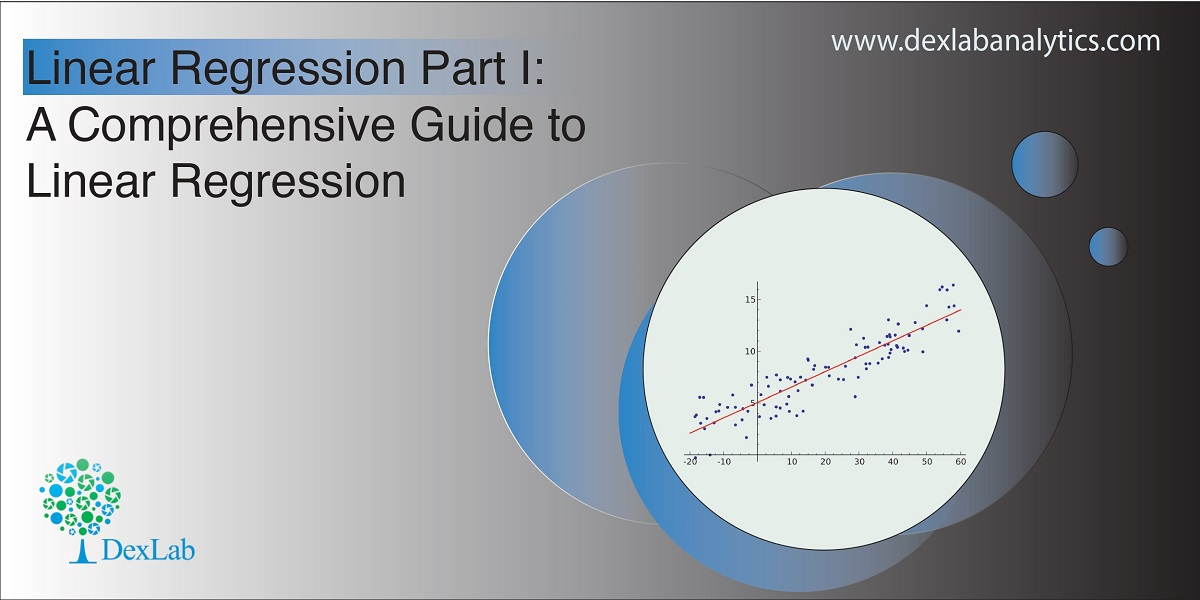Today’s blog explores another vital statistical concept Linear Regression, let’s begin. Linear regression is normally used in statistics for predictive modeling. It tries to model a relationship between two independent (explanatory variable) and dependent (explained variable) variables X and Y by fitting a linear equation (Y=bo+b1X+Ui) to an observed data.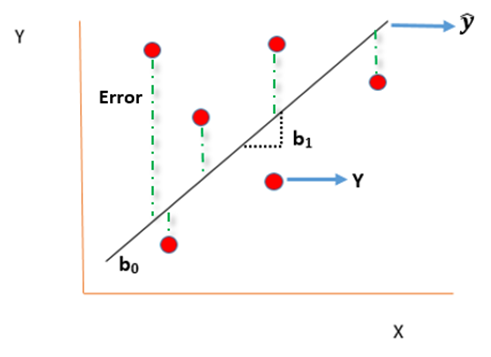#### Assumptions of linear regression

• Ui is a random real variable, where Ui is the difference between the observed dependent variable Y and predicted Y variable.
• The mean of Ui in any particular period is zero.
• The variance of Ui is constant in each period i.e for all values of X, Ui will show the same dispersion around their mean
• The variable Ui has a normal distribution i.e the value of Ui (for each Xi) have a bell shaped symmetrical distribution about their zero mean.
• The random terms of different observations are independent i.e the covariance of any Ui with any other Uj is equal to zero.
• Ui is independent of the explanatory variable X.
• Xi are a set of fixed values in the hypothesised process of repeated sampling which underlies the linear regression model.
• In case there are more than one explanatory variables then they are not perfectly linearly correlated.

Linear Regression equation can be written as: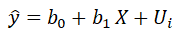Where,

is the dependent variable

X is the independent variable.

b0 is the intercept (where the line crosses the vertical y-axis)

b1 is the slope

Ui is the error term (difference between ) also called residual or white noise.#### Simple linear regression follows the properties of Ordinary Least Square (OLS) which are as follows:-

1. Unbiased estimator:- E()=b ie. an estimator is unbiased if its bias is 0; E() – b = 0
2. Minimum Variance:- An estimate is best when it has the smallest variance as compared to any other estimate obtained from other econometric method.
3. Efficient estimator:- When it has both the previous properties ie.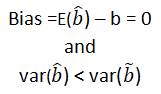4. Linear estimator
5. Best, Linear, Unbiased estimator (BLUE)
6. Minimum mean squared error (MSE) estimator:- It is a combination of the unbiasedness and minimum variance properties. An estimator is a minimum MSE estimator if it has the smallest mean square error.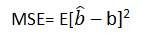With that the discussion on Linear Regression wraps up here, hopefully it cleared away any confusion you might have and helped you get a grasp on the concept. We have a video discussion on this same topic, which is attached below this blog, check it out for further reference.

Continue to track the DexLab Analytics blog to find informative posts related to Python for data science training.

.

## Regular Expression in Python Part III: Learn To Substitute With Re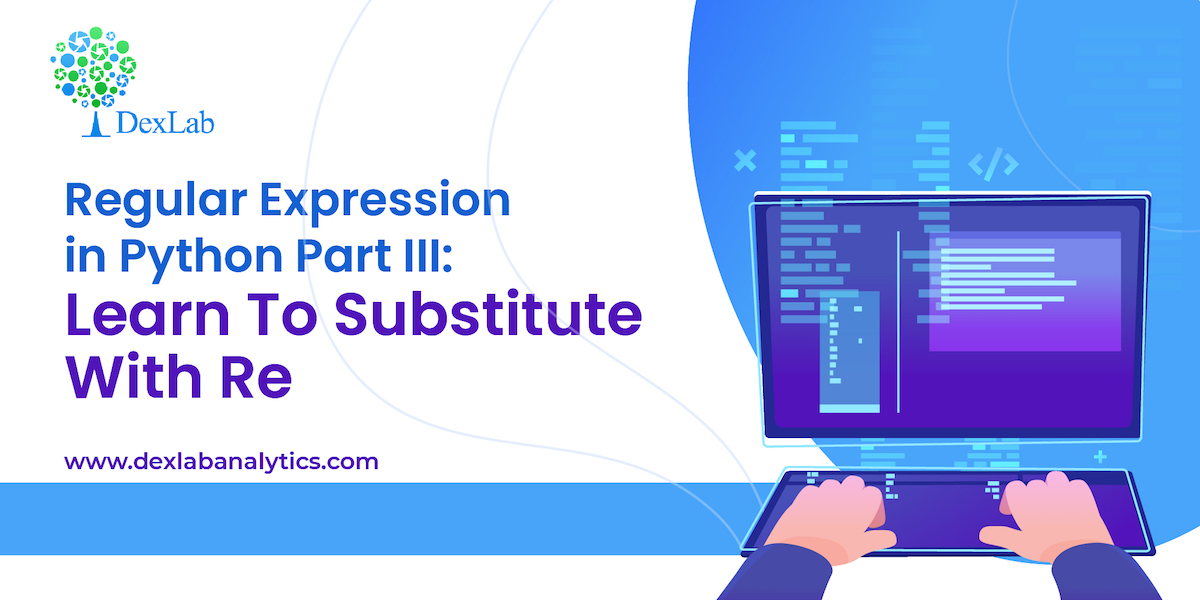This is the 3rd part of the ongoing Regex or, regular expression in Python series where we are discussing how to handle textual data. In the second part we introduced you to the re library and in this third segment, we are going to be discussing how to substitute characters or, words with re library.

Re library has a wide range of methods to deal with textual data, one such method is .sub() which helps us substitute alphabets or words based on the patterns we build. This method can be used with .match() method and .search() method, both having differences in the way they extract a pattern.

Difference between .match() & .search()

.match() :- This method extracts the required text only at the begging.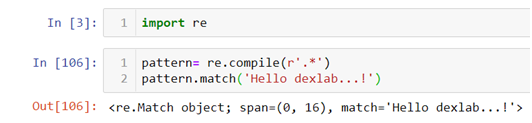.search() :-This can extract the required string from the entire text but only at the first occurrence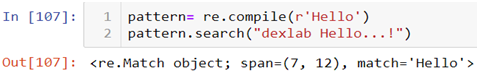In the above code you can see that even though the word “Hello” is in the middle of the text we are able to fetch it because of the special attribute of the .search() method. Here we are again using “Hello dexlab…!” as an example and .compile() is being used to create and apply our pattern.

Now suppose if we want to substitute the word “Hello” with another string “Hi” we will have to use .sub() method from the re library. But there are ways to use this method directly or indirectly.

First let’s see the direct method.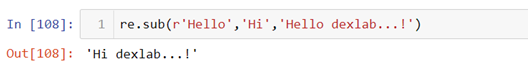In the above line of code we first mention what we want to substitute and with what and then we add the text.

Second way to do this is by first using .compile() method to build the pattern and then use that pattern to substitute the alphabet or word.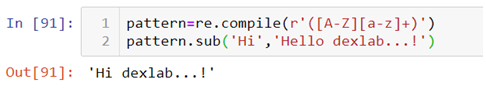The above pattern in the .complie() method states that there is a word with the first alphabet in uppercase combined with lowercase alphabets to be substituted with the word “Hi”. This pattern can match any string with the same characteristics, for example:-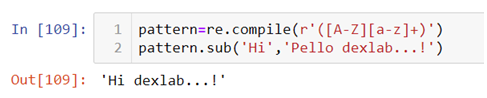Look at the text used in the .sub() method, now instead of “Hello” we have “Pello” with the same characteristics substituted with the word “Hi”. But one must not forget this pattern can also be used in the .sub() method directly and the use of .compile() method is optional. .compile() method is used only to create an object based code.

So, this wraps up the discussion on how to substitute characters or, words with re library. Hopefully, you found this blog informative, if you wish to find more Python Certification course topics, keep following the Dexlab Analytics blog.

.

## Regular Expression in Python Part II: Using re library for textual data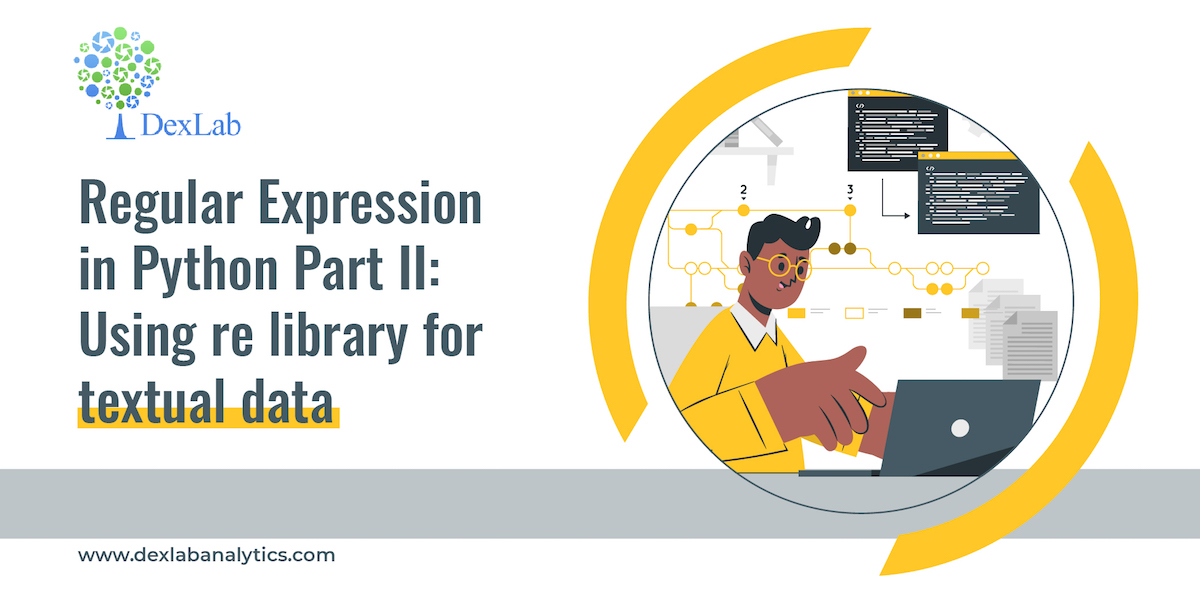This is the second part of the Regex series, where we will be continuing on by introducing you to another library in python, the re library. The previous blog introduced you to the Regex library in Python, and you learned about meta-characters and literals which could be used for creating patterns. This particular segment is about introducing the re library to help you with textual data analysis.

Re library in python holds the key to deal with all the problems relating to textual data analysis. This library provides a range of methods that can help you build patterns and extract or substitute the desired string. For example, suppose you want to change all the negative words to positive in a novel, for that all you need to have is a soft copy of the same and then you can import the re library and use its predefined methods first to make a pattern to extract the words and then substitute it to make the required changes.

Here one such method which we are going to use today from the re library is .compile() combined with .match() method to build and extract the pattern with the help of literals and meta-characters explained in the previous blog.

#### .compile() and .match() Methods

.complie() is used to build the pattern. You can use the meta-characters and literals within the parenthesis to build the pattern of the word which you want to extract or change. This is practically the first step without which not much can be done in re library. But why do we need a pattern and what is it that makes it necessary? To answer this question I am going to use few steps:-

1. First thing to do is to observe the word or the string and see what is it that makes that specific word or words which you want to extract different from the rest of the text i.e. is the word a combination of digit or alphabet or special character, is there a special character before and after the word etc.
2. Now recall all the meta-characters we have studied so far.
3. Combine them with the .complie() method which basically helps us bring the meta-characters together.
4. Now all there is left for us to do is apply the pattern on the text.

Therefore knowledge of meta-characters is necessary to form patterns to manipulate your textual data.

Now let’s see how to import re library and practically solve few of the Regex questions.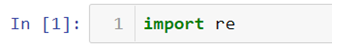Use the above code to import the Regex library.

Question 1: How to make a pattern to extract the entire string “Hello dexlab…!!”?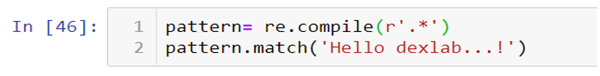In the above code we are using a combination of. (period)and * where

• . (period) means match alpha-numeric or special character
• * means match anything zero or more times

When combined together this means that you can match alpha-numeric or special characters zero or more times. Therefore the end result is:-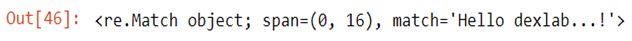.match()method has a special property that it can match anything only at the beginning of the line. Suppose I want to extract “Hello” from a string, .match()method will only work when word is at the 0 index.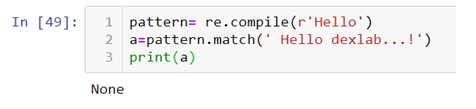Question: How to extract and match only special characters?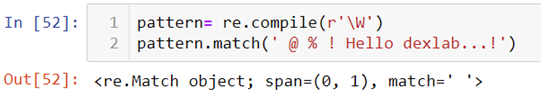The above code is matching only the space which is at the 0th index but not all the simultaneous special characters. To make a pattern that can match all the special characters we can use *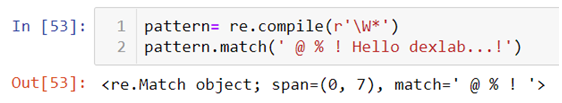You can try ? and + to check the difference it makes on the output.

Question: How to extract numbers and special characters?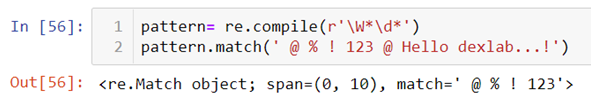Now you must be wondering why the above code did not recognize the special characters after the numbers like @ and space. Here you must remember that the output you get is based on the pattern you make. In the above code we mentioned nothing that matches anything after the numbers. So we further need to expand our line of codes.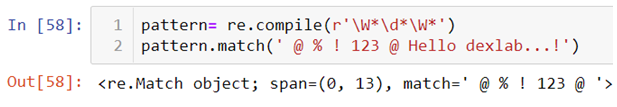Question: How to extract only the output?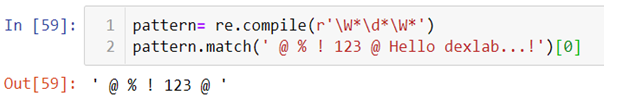You can use the slice operator [] to extract only the text by using 0 index.

You must have picked up the fundamentals of the re library from the blog, watch the video attached below to follow the tutorial step by step. Follow the Regex series to gain expertise in textual data handling. Dexlab Analytics blog has more interesting and informative posts on Python Programming training.

.

## Regular Expression in Python Part I: Introducing Regex Library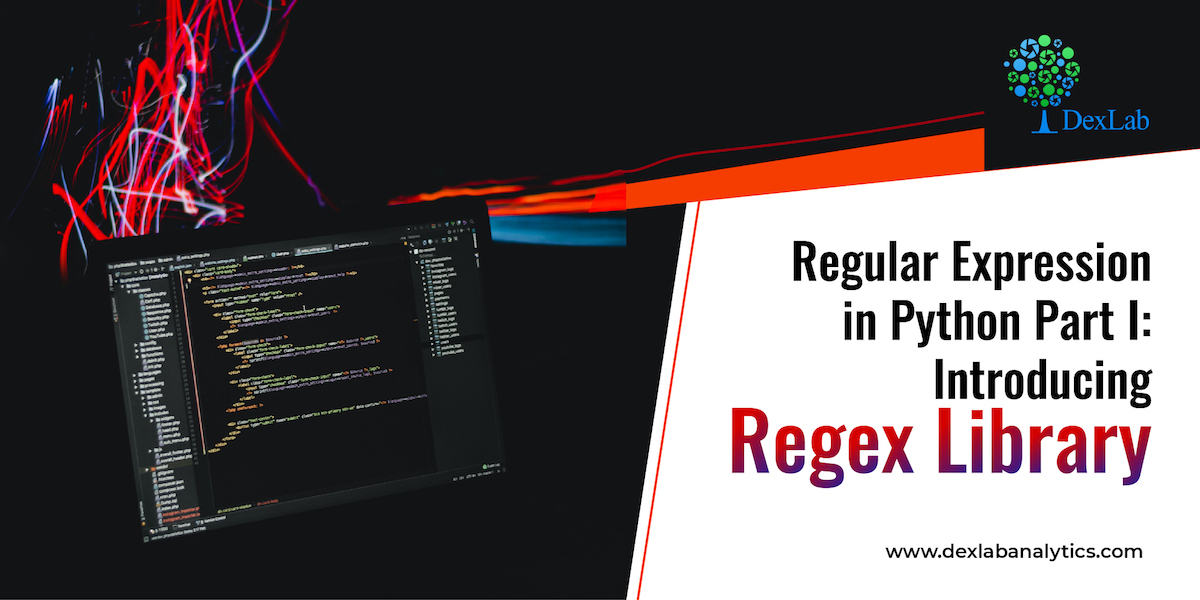Python is a versatile programming language and it has a rich library. In the visualization series we introduced you to different libraries used for data visualization purposes. Now, we introduce you to the Regex library in Python for handling textual data.

In Python to perform pattern recognition on textual data Regex is a library that provides a range of methods which when used with right pattern gives us the desired results. For example, if you want to change the spelling of colour to color in your text you can easily do so with the help of a given method provided that you form the pattern correctly.

#### Type of textual data in Regex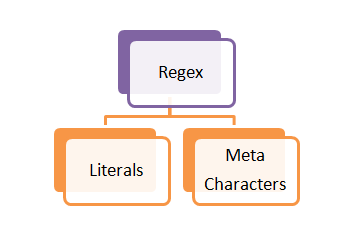Literals:- In Python literals are the characters or words with their original meaning intact like the word dog means a literal dog and there is no hidden meaning behind that word.

Meta-characters:- These are the words or characters which hold special meaning for example \n means a new line or \t means tab separated values.

#### Given below are few of the meta-characters used in python with their meanings:-

\d – Matches a digit .i.e. \d= 1 ,\d\d= 23, \d\d\d = 345

\w – Matches alpha-numeric characters i.e. \w= 1, \w= a, \w\w= a1

\W– Matches special characters i.e. \W= %

Dog[ogn]– Matches a single character within the square bracketsi.e. Dogo, Dogg, Dogn

Dog(ogn) – Matches the entire string within the parenthesisi.e. Dogogn

Dog(ogn|aaa)– Matches either ogn or aaa i.e. Dogogn or Dogaaa

*– Matches 0 or more characters i.e. tre* = tree, tre*= tr, tre*= treeeeee

?– Matches 0 or 1 character i.e. colou?r= color, colou?r= colour

+ – Matches 1 or more character i.e. tre+= tree, tre+= treee, tre+≠tre

. – Matches alpha-numeric or special characters but only one time i.e. tre.= tree, tre.= tre#, tre.=tre1, tre.≠tre#1

The above meta-characters alone or in combination are used to form a pattern  which then are used for text mining for example tre.* means match anything 0 or more times that means now we can match tre#1 or tre.

Hopefully you found the discussion on Regex library helpful and at the end of it you must have become familiar with the way this particular library works. To learn more about python for data analysis, keep on exploring Dexlab Analytics blog, where you will always find informative posts.

.

+91 931 572 5902